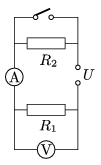Mathematical and Physical Journal
for High Schools
Issued by the MATFUND Foundation
 Already signed up? New to KöMaL?

#Problem P. 4195. (November 2009)

P. 4195. The voltage of the power supply of the circuit shown in the figure is 15 V. When the switch is open the voltmeter reads 10 V and the ammeter reads 200 mA.

a) Find the resistances of all the resistors.

b) What are the readings of the meters when the switch is closed?

c) Find the dissipated power at each resistor when the switch is open and when it is closed.(3 pont)

Deadline expired on December 10, 2009.

Sorry, the solution is available only in Hungarian. Google translation

Megoldás. $\displaystyle a)$ $\displaystyle R_{1}=50~\Omega,$ $\displaystyle R_{2}=25~\Omega.$

$\displaystyle b)$ 300 mA, illetve 15 V.

$\displaystyle c)$ Nyitott kapcsolóállásnál $\displaystyle P_{1}=2~$W, $\displaystyle P_{2}=1~$W; zárt állásnál $\displaystyle P_{1}=4,5~$W, $\displaystyle P_{2}=0.$

### Statistics:

 200 students sent a solution. 3 points: 111 students. 2 points: 59 students. 1 point: 19 students. 0 point: 9 students. Unfair, not evaluated: 2 solutionss.

Problems in Physics of KöMaL, November 2009